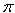SEARCH HOMEMath Central Quandaries & QueriesQuestion from James, a student: My question goes: A silo is to be constructed and surmounted by a hemisphere. The material of the hemisphere cost twice as much as the walls of the silo. Determine the dimensions to be used of cost is to be kept to a minimum and the volume is fixed. Obviously the answer is going to be some kind of ratio of height to radius. First I determined the formula for the cost and volume, and both height and radius in terms of both volume and cost. I have C=2piRH+4piR^2, V=HpiR^2+(2piR^3)/3 and all the formulas for H and R that can be derived from these. From here I have put cost in terms of volume, radius, and height in nearly every combination possible, like C=[(9V-(2/3)piR^3)/2R]+[(V-piR^2H)/6R]. I can substitute all my formulas for H and R so that I only have one variable and set the derivative of that equal to zero and solve for H or R but even if I do that there is no way to find the other variable. And I can't just magically take the derivative of C with respect to H and R. I think I have to find the derivative in terms of either H or R, and then with that form a ratio of H and R with the equations for C and V, but I just can't seem to pull that off on paper.Hi James,

I agree with what you have done in developing expressions foe the volume and cost with one small change. You don't know the costs of the materials just that one material is twice as expensive as the other. Suppose that the material for the walls costs $k per square unit then the material for the hemisphere costs$2k per square unit. Then the cost of materials is

C = 2kRH + 4kR2

and the volume is

V =HR2+(2R3)/3

As you pointed out the challenge now is to express C as a function of one of the variables R and H and then differentiate, keeping in mind that k and V are constants. The key is the equation for the volume which can be writtenHR2= V - (2R3)/3

and hence

H = [V - (2R3)/3]/[R2]

Substitute this value for H in the expression for the cost and the cost then becomes a function of R alone.

PennyMath Central is supported by the University of Regina and The Pacific Institute for the Mathematical Sciences.